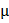# Consider a tank made of glass (reiractive index 1.5) with a thick bottom.  It is filled with a liquid of refractive index., A student finds that, irrespective of what the incident angle i(see figure0 is for a beam of light entering the liquid, is never reflected from the liquid glass interface happen, the minimum value ofis:a.b.c.d.## Question ID - 50056 :- Consider a tank made of glass (reiractive index 1.5) with a thick bottom.  It is filled with a liquid of refractive index., A student finds that, irrespective of what the incident angle i(see figure0 is for a beam of light entering the liquid, is never reflected from the liquid glass interface happen, the minimum value ofis:a.b.c.d.3537

c < ib

Here ib is “brewester angle”

And c is critical angle

sinc+sinib

Since tan ib==<sin ib=< 1.52+(1.5)2< (1.5)2<Slab=1.5

Next Question :
 The ratio of the height of a room to its semi-perimeter is 2:5. It costs Rs. 260 to paper the walls of the room with paper 50 cm wide at Rs. 2 per m allowing an area of 15 sq. m for doors and windows. The height of the room is (a) 2.6 m (b) 3.9 m (c) 4 m (d) 4.2 m